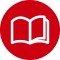MATH110-22S1 (C) Semester One 2022

# Foundations of Applied Mathematics and Statistics

15 points

Details:
 Start Date: Monday, 21 February 2022 End Date: Sunday, 26 June 2022
Withdrawal Dates
Last Day to withdraw from this course:
• Without financial penalty (full fee refund): Sunday, 6 March 2022
• Without academic penalty (including no fee refund): Sunday, 15 May 2022

## Description

Introduction to trigonometry and algebra. Emphasis on solving problems relevant to design, physical, life and earth sciences as well as to commerce and the humanities. An introduction to the ideas, techniques and applications of statistics and probability.

This course provides a foundation of mathematics and practical techniques for using, summarizing and visualizing data from a user’s point of view. The emphasis is on sensible and correct use or interpretation of mathematical and data analysis tools in real-life problems. The course assumes elementary levels of competence in mathematics. Microsoft Excel™ will be used as a tool within the course.
The emphasis of this course is on applying the methods covered in the course to problems, selecting sensible techniques, following the methodology and interpreting the results.  Practical Excel skills are taught as a tool for performing calculations and for analysing and visualizing data.  Applications to commerce, the social sciences, humanities, science and engineering are considered.

## Learning Outcomes

• Students who pass this course will be able to:
• Use equations and formulae that describe various physical phenomena encountered in science and engineering
o use basic algebra to simplify expressions and rearrange equations
o use the rules of exponents
o solve linear equations and non-linear equations
o interpret and solve inequalities
o model linear and quadratic relationships
o graph and interpret linear, quadratic, exponential and logarithmic models
o apply equations used to calculate the area and volume of physical objects
o apply trigonometry to simple geometric calculations
o interpret the slope and area under a curve in an applied context (such as acceleration, velocity, displacement)
o calculate an approximation to the area under a curve
• Calculate probabilities using Venn diagrams and tabulated data
o apply the additive rule, complements, and the multiplicative rule for independent events
• Perform calculations using factorials, permutation, & combinations.
• Interpret the slope and intercept of a linear model between two variables
• Use Excel to:
o perform numerical calculations.
o produce data visualizations including bar and pie charts, line charts and scatter plots
o perform data analysis including data summaries (descriptive statistics), and two-way table analyses using Pivot Tables.
o fit a linear model to bivariate data using simple linear regression.

This course will provide students with an opportunity to develop the Graduate Attributes specified below:Critically competent in a core academic discipline of their award Students know and can critically evaluate and, where applicable, apply this knowledge to topics/issues within their majoring subject.

## Assessment

Lab quizzes (6 @ 5% each) 30%
Assignments (2 @ 8% each) 16%
Final Examination 54%

To obtain a passing grade in this course you must obtain at least 50% overall and at least 50% in each part of the final examination (mathematics and statistics).

## Textbooks / Resources

Barton, David , Cox, David; Essential maths and stats : for higher education ; Pearson, 2013.

Lock, Robin H. et al; Statistics : unlocking the power of data ; Second edition; Wiley, 2017.

## Indicative Fees

Domestic fee \$802.00

International fee \$4,563.00

* All fees are inclusive of NZ GST or any equivalent overseas tax, and do not include any programme level discount or additional course-related expenses.

For further information see Mathematics and Statistics .

## All MATH110 Occurrences

• MATH110-22S1 (C) Semester One 2022